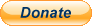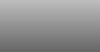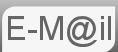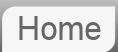# Answer to Riddle #8: Remainders

8. What is the smallest positive integer that leaves a remainder of 1 when divided by 2, remainder of 2 when divided by 3, a remainder of 3 when divided by 4, and so on up to a remainder of 9 when divided by 10?

The answer to this, (along with #9 & #16,) was sent to me by Dave Blackston who visited me from fark.com (then corrected by Daya Chand)...

The key to this is to realise that if a number N (eg 104) is to leave a remainder, say 4 when divided by 5 then N + 1 (ie 105) is exactly divisible by 5, similarly if a number (eg 87) is to leave a remainder 7 when divided by 8 then N + 1 (ie 88) is exactly divisible by 8:-

Therefore in our question N + 1 is divisible by 2, 3, 4, 5, 6, 7, 8, 9 & 10

There is a tool here you can use to try different values of N

Note: no information is sent to me, the calculation is done entirely locally, on your computer.
Meaning N+1 is 2000

N+1 has the following factors:
2  3  4  5  6  7  8  9  10  All Factors

The lowest value for N + 1 is 2520, which means the lowest value for N is:-

2519

## How do we arrive at N + 1 = 2520?

As stated N + 1 is divisible by 2, 3, 4, 5, 6, 7, 8, 9 & 10 so clearly one solution would be 10! (ie 10*9*8*7*6*5*4*3*2 = 3628800 for N +1) but this is not the lowest possible. Follow the logic below...

N + 1 must be a multiple of 2

N + 1 must be a multiple of 4 but if it is a multiple of 2 & 4 it is necessarily a multiple of 8

N + 1 must be a multiple of 5 but if it is a multiple of 2 & 5 it is necessarily a multiple of 10

N + 1 must be a multiple of 6 but if it is a multiple of 2 & 9 (18) it is necessarily a multiple of 6

N + 1 must be a multiple of 7

N + 1 must be a multiple of 9

Hence 2 x 4 x 5 x 7 x 9 = 2520 is a multiple of 2, 3, 4, 5, 6, 7, 8, 9 & 10

Mathematically the process we are dealing with here is known as lowest or least common multiple.

Google got the closest, but it only gets a 5/10

If you're curious what Bard made of this puzzle...

If you're curious what ChatGPT made of this puzzle...

© Nigel Coldwell 2004 -  – The questions on this site may be reproduced without further permission, I do not claim copyright over them. The answers are mine and may not be reproduced without my expressed prior consent. Please inquire using the link at the top of the page. Secure version of this page.PayPal
I always think it's arrogant to add a donate button, but it has been requested. If I help you get a job though, you could buy me a pint! - nigel